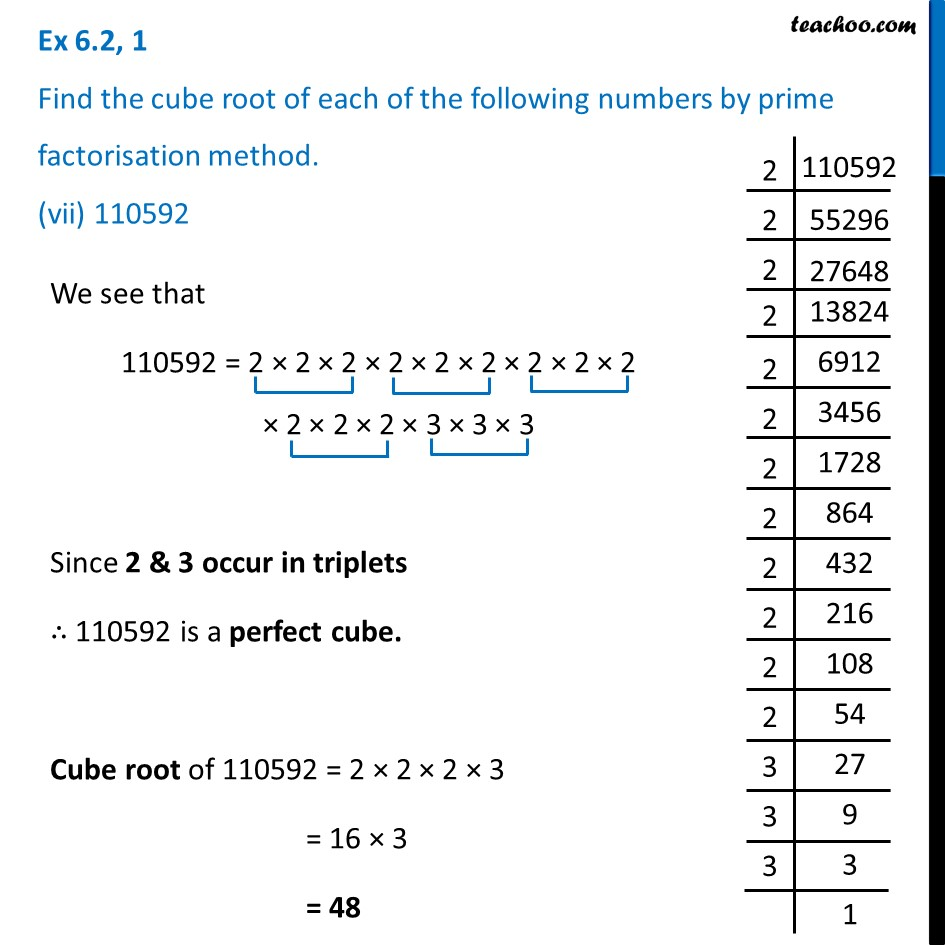Ex 6.2

Chapter 6 Class 8 Cubes and Cube Roots
Serial order wiseLearn in your speed, with individual attention - Teachoo Maths 1-on-1 Class

### Transcript

Ex 6.2, 1 Find the cube root of each of the following numbers by prime factorisation method. (vii) 110592We see that 110592 = 2 × 2 × 2 × 2 × 2 × 2 × 2 × 2 × 2 × 2 × 2 × 2 × 3 × 3 × 3 Since 2 & 3 occur in triplets ∴ 110592 is a perfect cube. Cube root of 110592 = 2 × 2 × 2 × 3 = 16 × 3 = 48